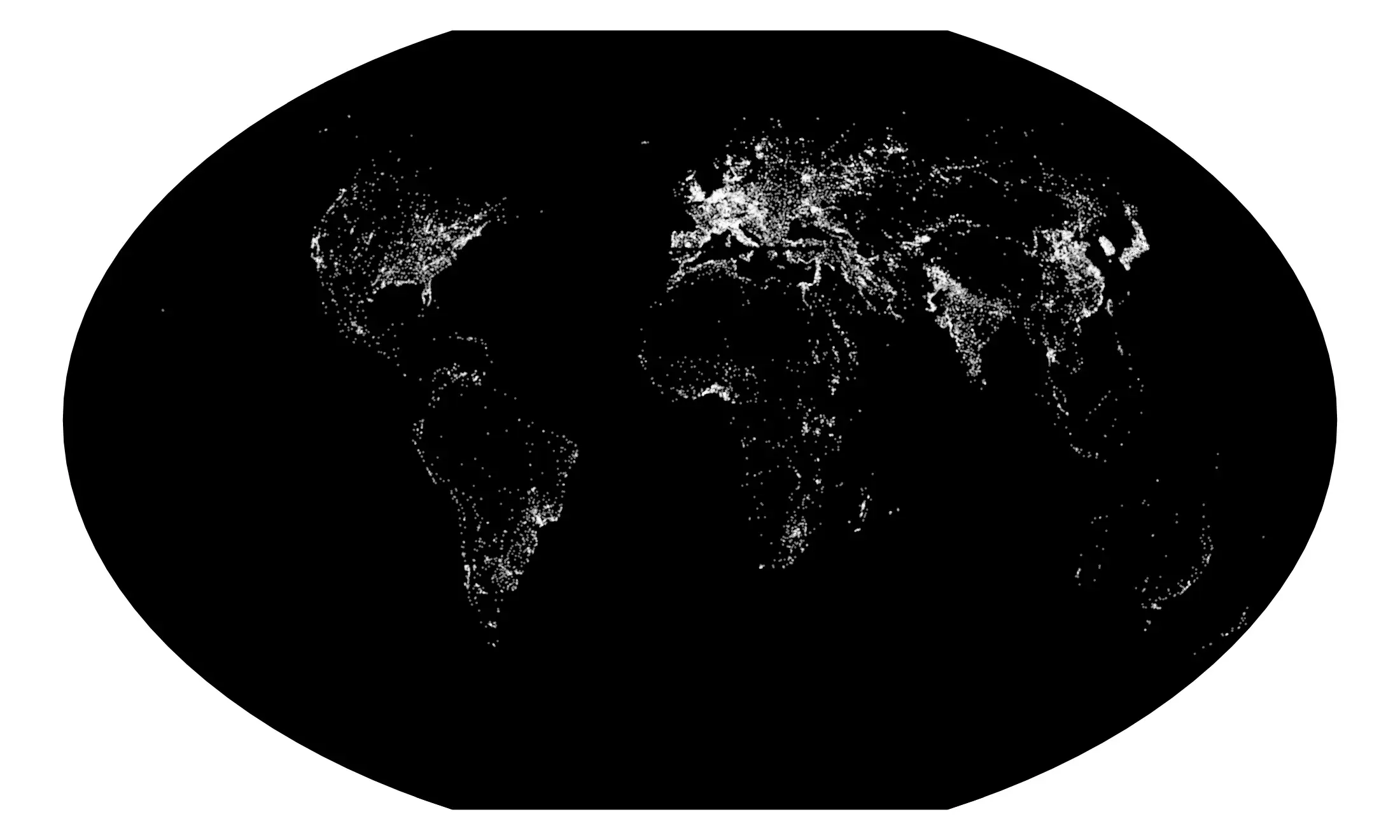sylvain durand

# Spatial Data Analysis with R

If R language has already become a reference in statistical analysis and data processing, it may be thanks to its hability to represent and visualize data. They can be used in order to visualize spatial data in the form of cartographic representations which, combined with its other features, makes it an excellent geographic information system. This article sets out to show, through the provision of relevant example, how R can handle spatial data by creating maps.

### Prerequisite

Once R is installed on your computer, few libraries will be used: `rgdal` allows us to import and project shapefiles, `plotrix` creates color scales, and `classInt` assigns colors to map data. Once the libraries installed with `install.packages`, load them at the beginning of the session:

``````library('rgdal')      # Reading and projecting shapefiles
library('plotrix')    # Creating color scales
library('classInt')   # Assigning colors to data
``````

Graphics will be plot using R base functions. `ggplot2` is an alternative, but it seems less relevant here: longer and less legible code, unability to plot holes inside polygones, `fortify` and ploting can last much longer.

## Blank France map

The `rgdal` library provides `readOGR()` in order to read shapefiles. `dsn` must contain the path where shapefiles are located, and `layer` the shapefile name, without extension. `readOGR` reads `.shp`, `.shx`, `.dbf` and `.prj` files. Departements of France are given by Geofla:

``````# Reading departements

# Reading departements boundaries in order to plot France boundaries
bounderies <- bounderies[bounderies\$NATURE %in% c('Fronti\xe8re internationale','Limite c\xf4ti\xe8re'),]
``````

In order to show neighbouring countries, we will use data provided by Natural Earth. We will select Europe countries only:

``````# Reading country and selecting Europe
europe <- europe[europe\$REGION_UN=="Europe",]
``````

### Projection and plot

The map will use the French official projection “Lambert 93”, already declared in the Geofla `.prj` files. `spTransform` will be used for the European coutries.

Then, we will first plot French boundaries, in order to center the map on France. Borders colors are defined in `border`, their tickness in `lwd` and the filling color in `col`.

``````# Projection
europe <- spTransform(europe, CRS("+init=epsg:2154"))

# Plot
pdf('france.pdf',width=6,height=4.7)
par(mar=c(0,0,0,0))

plot(bounderies,  col="#FFFFFF")

dev.off()
``````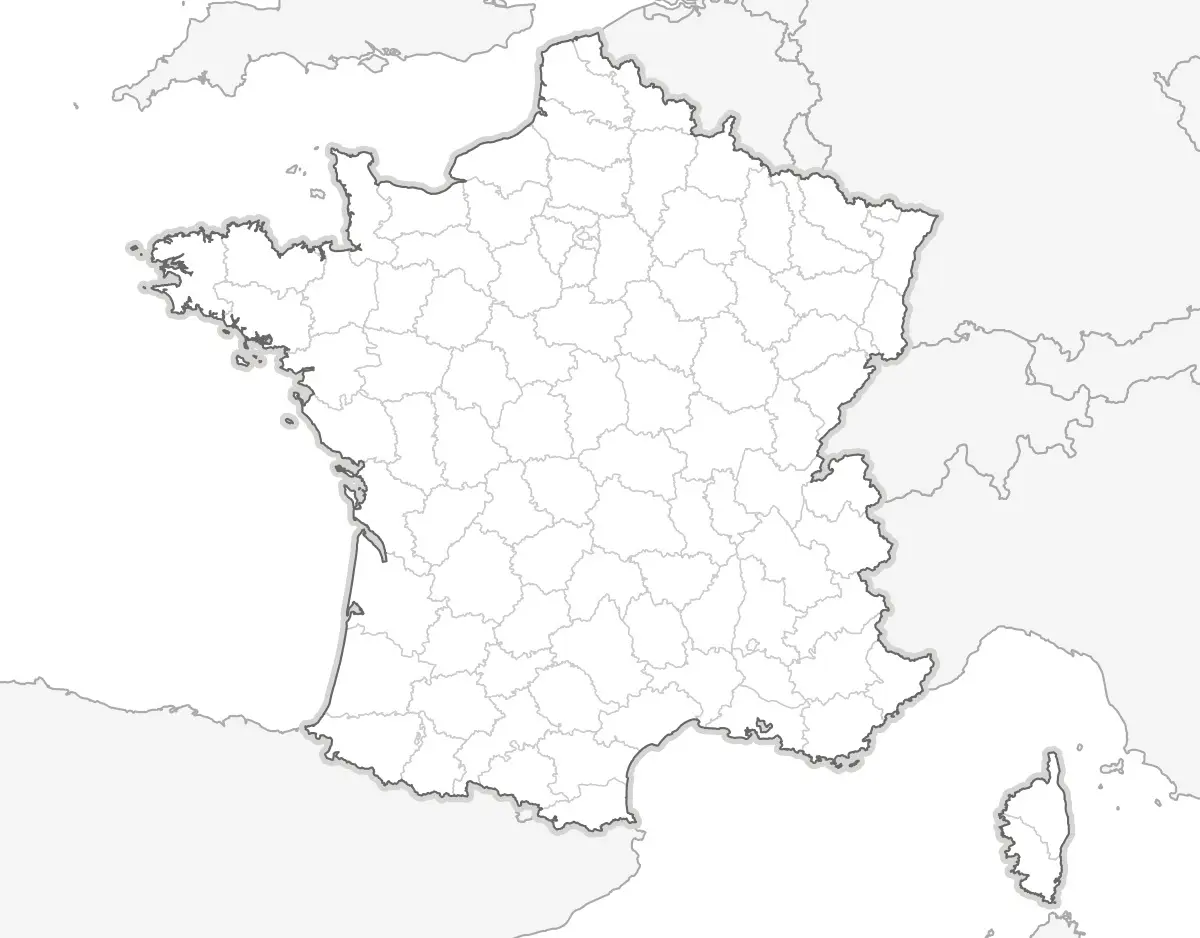## Visualizing a data: population density

The very large number of communes (the smallest administrative level in France) gives us excellent spatial data. Geofla provides us their bounderies, population and area. So we will plot population density:

``````# Reading shapefile
# Calculate density
communes\$DENSITY <- communes\$POPULATION/communes\$SUPERFICIE*100000
``````

### Color scale

In order to create a color scale, we will assign shades of blue to each percentile. `classIntervals` calculates percentiles, `smoothColors` create the blue scale, and `findColours` assigns blues depending on each commune depending on their population density. Then, we create a legend, with only five colors.

``````# Color scale
col <- findColours(classIntervals(
communes\$DENSITY, 100, style="quantile"),
smoothColors("#0C3269",98,"white"))
# Legend
leg <- findColours(classIntervals(
round(communes\$DENSITY), 5, style="quantile"),
smoothColors("#0C3269",3,"white"),
under="less than", over="more than", between="–",
cutlabels=FALSE)
``````

### Plot

We can now plot the map. In order to use an embedded font in the PDF, we will use `cairo_pdf()` instead of `pdf`:

``````# Exporting in PDF
cairo_pdf('densite.pdf',width=6,height=4.7)

# Ploting the map
plot(bounderies, col="#FFFFFF")

# Ploting the legend
legend(-10000,6387500,fill=attr(leg, "palette"),
legend=names(attr(leg,"table")),
title = "Density (p/km²):")

dev.off()
``````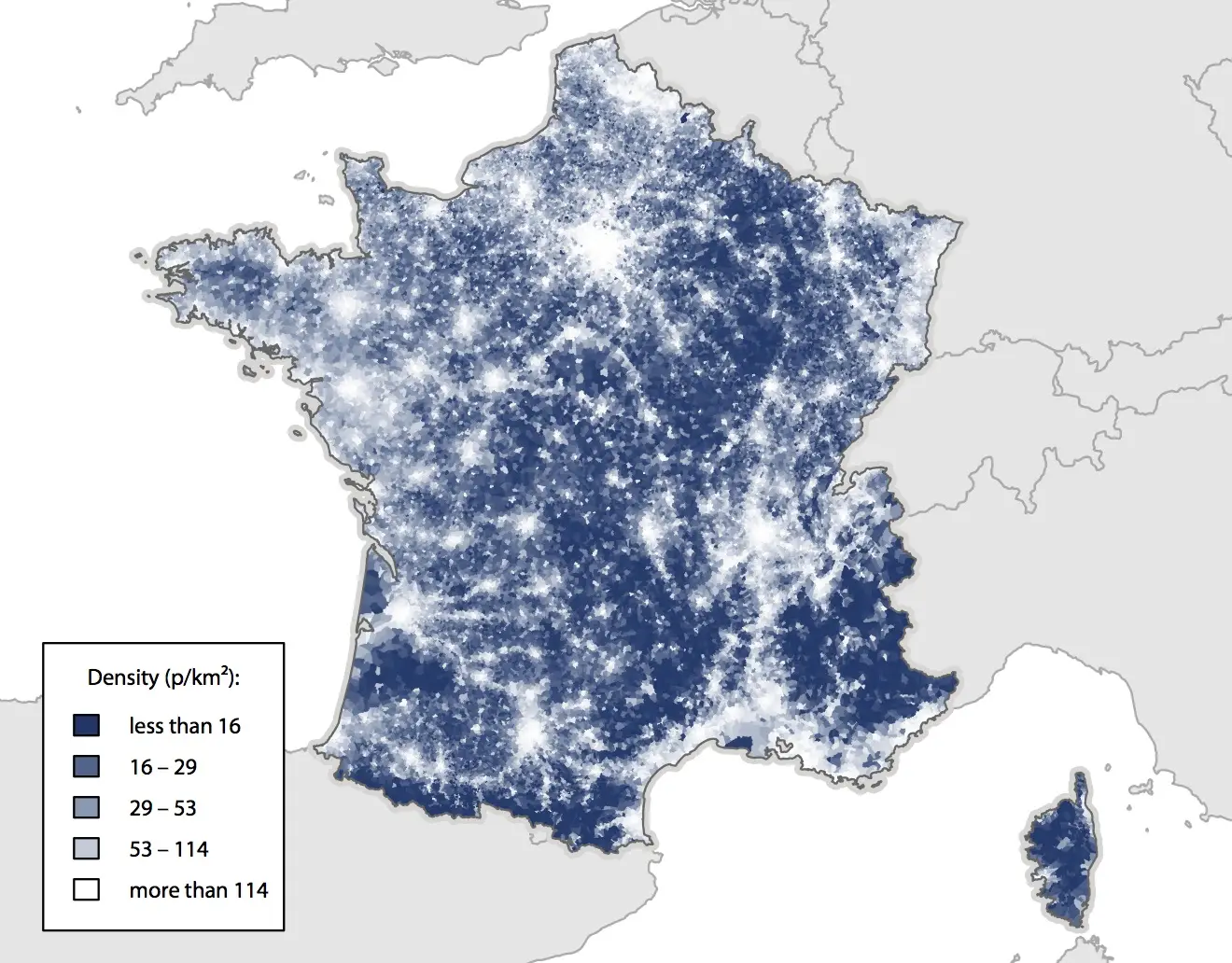## Visualizing an external data: incomes

The main value is to plot data provided by external files. We will plot the median taxable income per consumption unit.

Unfortunately, data is missing for more than 5 000 communes, due to tax secrecy. We can “cheat” in order to improve the global render by assigning to those communes the canton (a larger administrative level) median income, given in the same file.

``````# Loading communes data
communes <- merge(communes, incomes, by.x="INSEE_COM", by.y="COMMUNE")

# Merging data
communes <- merge(communes, cantons, by="CANTON", all.x=TRUE)

# Assigning canton median income to communes with missing data
communes\$REVENUS[is.na(communes\$REVENUS)] <- communes\$REVENUC[is.na(communes\$REVENUS)]
``````

### Color scale

We generate color scale and legend:

``````col <- findColours(classIntervals(
communes\$REVENUS, 100, style="quantile"),
smoothColors("#FFFFD7",98,"#F3674C"))

leg <- findColours(classIntervals(
round(communes\$REVENUS,0), 4, style="quantile"),
smoothColors("#FFFFD7",2,"#F3674C"),
under="moins de", over="plus de", between="–",
cutlabels=FALSE)
``````

### Plot

And we plot:

``````cairo_pdf('incomes.pdf',width=6,height=4.7)

plot(boundaries, col="#FFFFFF")

legend(-10000,6337500,fill=attr(leg, "palette"),
legend=gsub("\\.", ",", names(attr(leg,"table"))),
title = "Median income:")

dev.off()
``````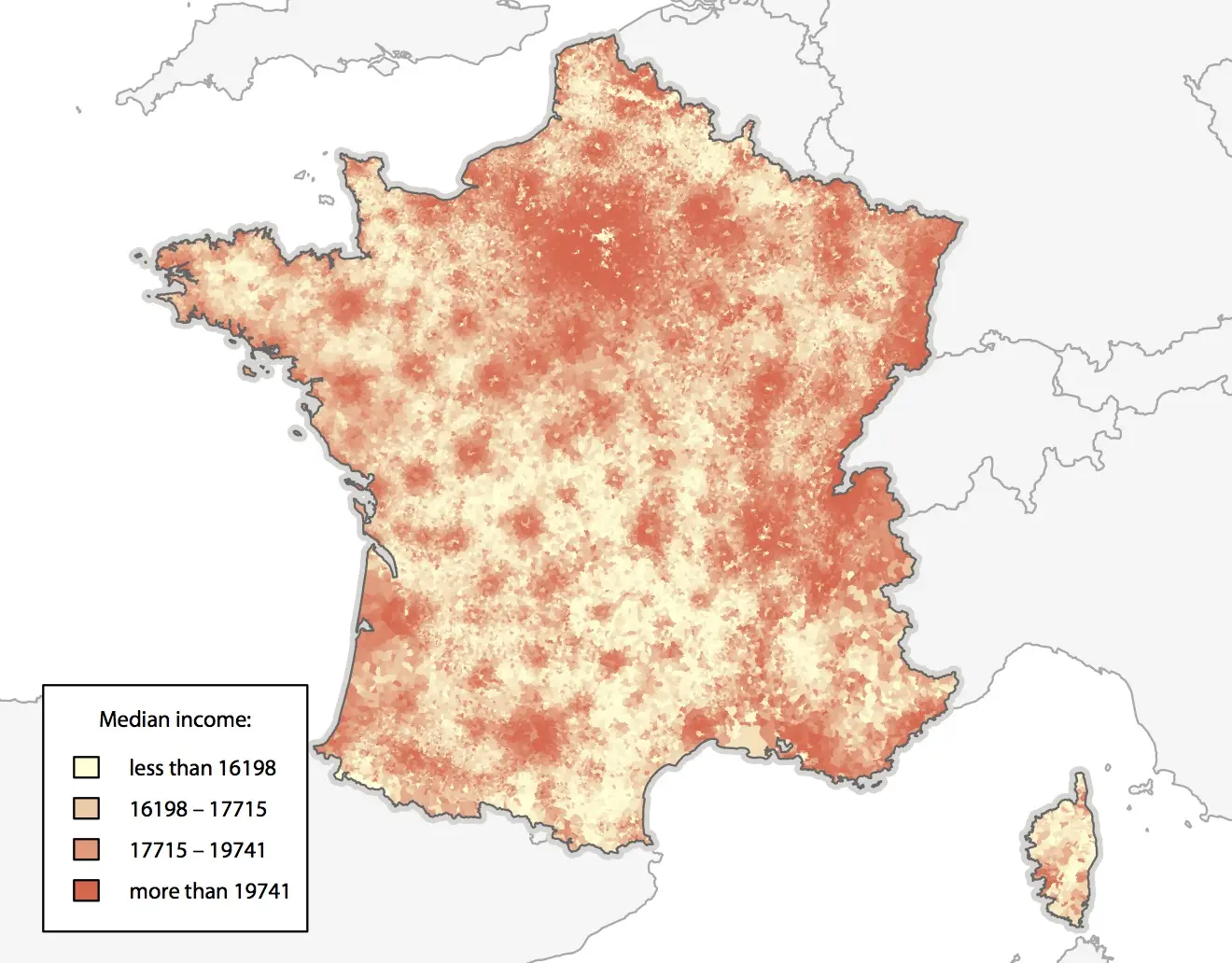## Visualizing map data: the road network

We can also add map data: cities, urban areas, rivers, forests… We will here plot the French road network, thanks to Route 500, from IGN:

``````roads <- readOGR(dsn="shp/geofla",  layer="TRONCON_ROUTE")

plot(boundaries,  col="#FFFFFF")

dev.off()
``````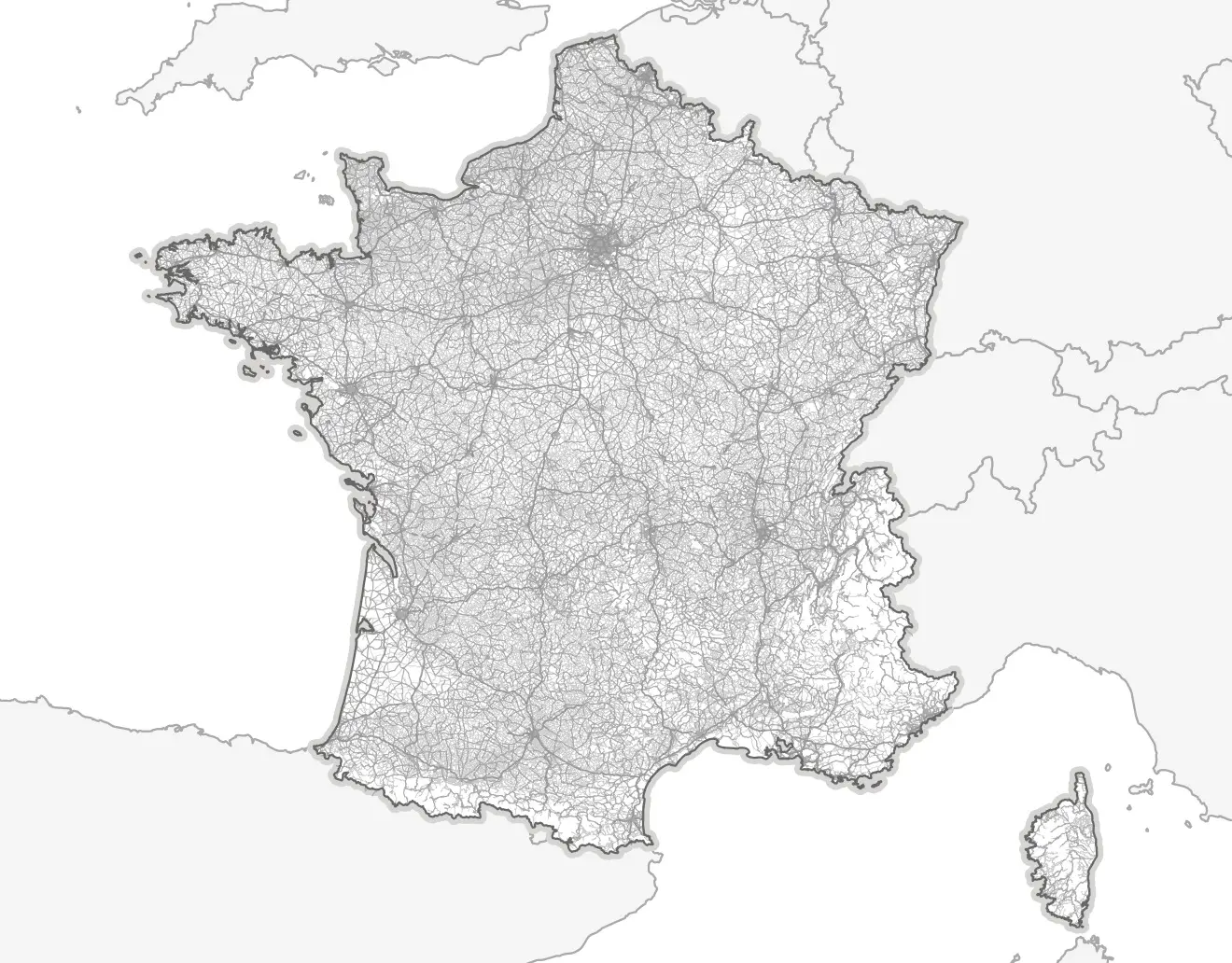## Blank world map

We will load the following Natural Earth:

``````# Loading Shapefiles
``````

### Projection and plot

We will use the Winkel Tripel projection:

``````# Winkel Tripel projection
countries   <- spTransform(countries,CRS("+proj=wintri"))
bbox        <- spTransform(bbox,  CRS("+proj=wintri"))
graticules  <- spTransform(graticules, CRS("+proj=wintri"))

# Ploting world map
pdf('world.pdf',width=10,height=6) # PDF export
par(mar=c(0,0,0,0))                # Zero margins

plot(bbox, col="white", border="grey90",lwd=1)

dev.off()                          # Saving file
``````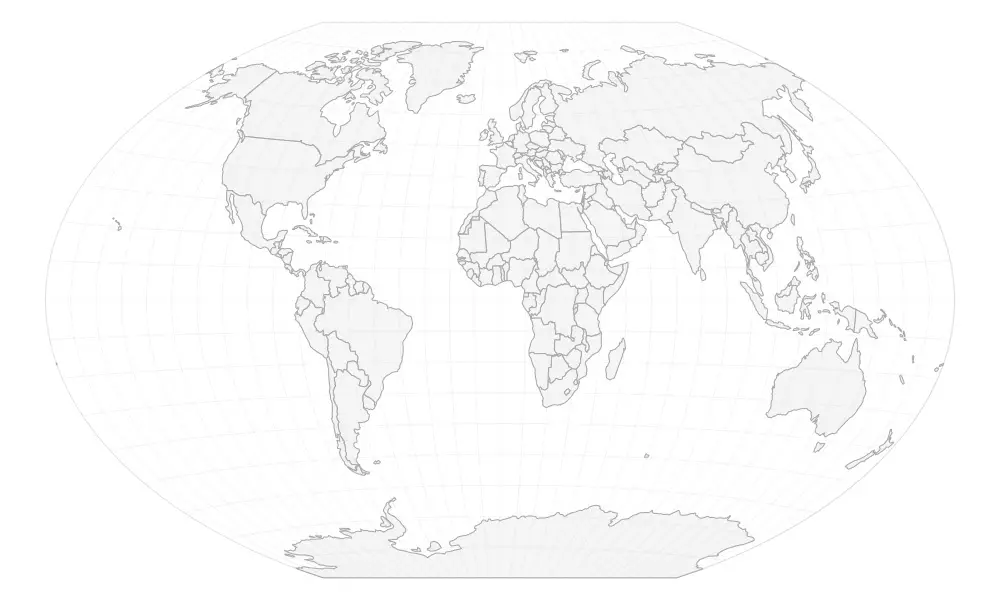## Visualizing data: Human Development Index

Most frequent usage consists of visualizing data with a color scale. Let’s plot the HDI, provided by the United Nations Develment Programme in a CSV file. The procedure is as described above:

``````# Loading data and merging dataframes
countries <- merge(countries, hdi, by.x="iso_a3", by.y="Abbreviation")

# Converting HDI in numeric
countries\$hdi <- as.numeric(levels(countries\$X2012.HDI.Value))[countries\$X2012.HDI.Value]

# Generating color scale and assigning colors
col <- findColours(classIntervals(countries\$hdi, 100, style="pretty"),
smoothColors("#ffffe5",98,"#00441b"))

# Assigning grey to missing data
col[is.na(countries\$hdi)] <- "#DDDDDD"

# Generating legend
leg <- findColours(classIntervals(round(countries\$hdi,3), 7, style="pretty"),
smoothColors("#ffffe5",5,"#00441b"),
under="less than", over="more than", between="–", cutlabels=FALSE)

# Ploting
cairo_pdf('hdi.pdf',width=10,height=6)

plot(bbox, col="white", border="grey90",lwd=1)

legend(-15000000,-3000000,fill=attr(leg, "palette"),
legend= names(attr(leg,"table")),
title = "HDI in 2012:")
dev.off()
``````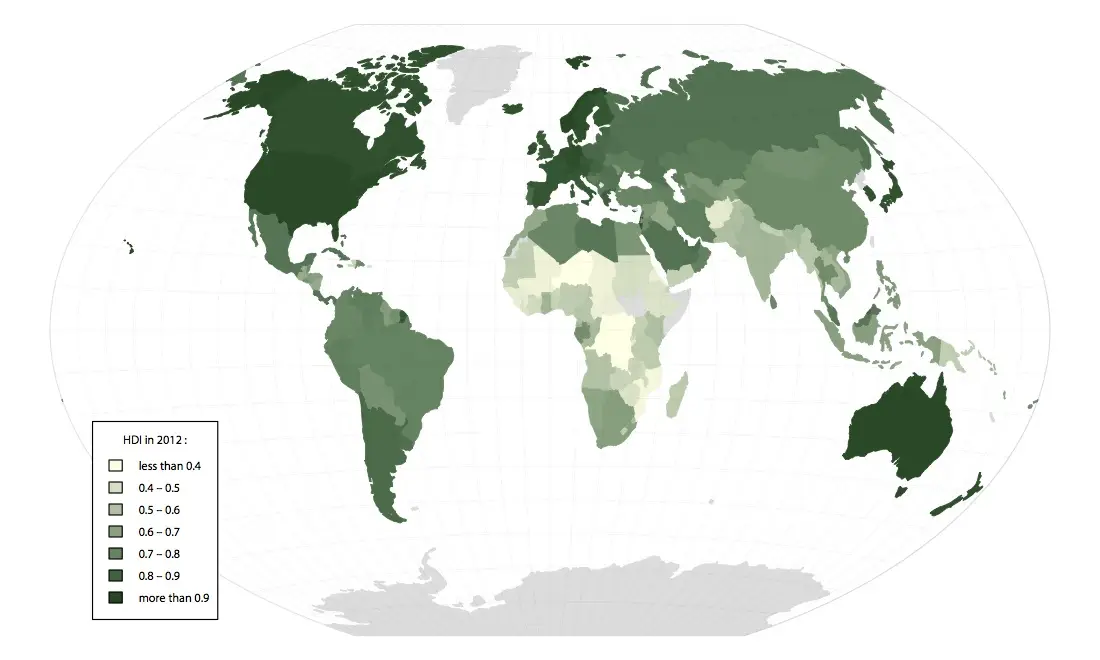## Circles visualization: most populated cities

An other kind of visualization is given by circles. Population of most populated cities is provided by Natural Earth:

``````# Loading shapefile
cities <- spTransform(cities, CRS("+proj=wintri"))
``````

### Circle size

The data shall be proportionate to the circles areas, not the radius; so the radius is the square root of the population:

``````# Calculating circles radius
``````

### Plot

We plot the map:

``````pdf('cities.pdf',width=10,height=6)
par(mar=c(0,0,0,0))

plot(bbox, col="white", border="grey90", lwd=1)
points(cities, col="#8D111766", bg="#8D111766", lwd=1, pch=21, cex=cities\$radius)

dev.off()
``````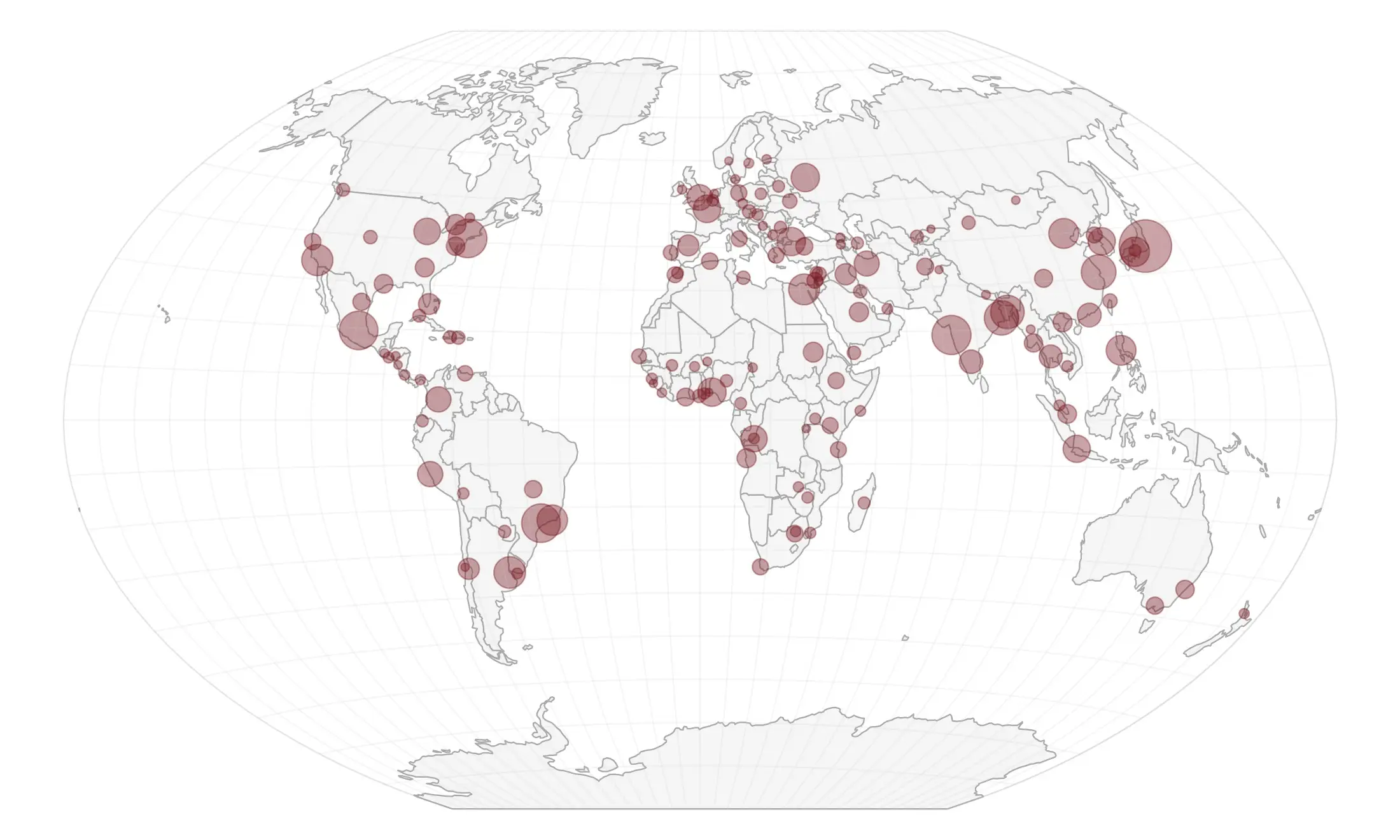## Visualizing map data: urban areas

Natural Earth provides urban areas shapefiles, derived from satellite data. Let’us map them with night lights colors:

``````areas  <- readOGR(dsn="shp/ne/cultural",layer="ne_10m_urban_areas")
areas <- spTransform(areas, CRS("+proj=wintri"))

pdf('areas.pdf',width=10,height=6)
par(mar=c(0,0,0,0))
plot(bbox, col="#000000", border="#000000",lwd=1)